The tool Create UMI Reads from Grouped Reads generates a single consensus read, called a UMI read, from reads which belong to the same group, as determined by the Calculate Unique Molecular Index Groups tool. The consensus reads are placed in a read mapping at the location of the original reads. Therefore, the output of the tool is a read mapping of generated UMI reads.

The tool can be found in the Toolbox here:

Toolbox | Biomedical Genomics Analysis (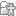) | UMI Tools () | Create UMI Reads from Grouped Reads (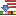)

In the first dialog (figure 4.4), select a read mapping of the original reads with UMI annotations that was previously handled with the Calculate Unique Molecular Index Groups tool.Figure 4.4: Select a read mapping of the original reads with UMI annotations.

The second dialog of the wizard (figure 4.5) offers the following options: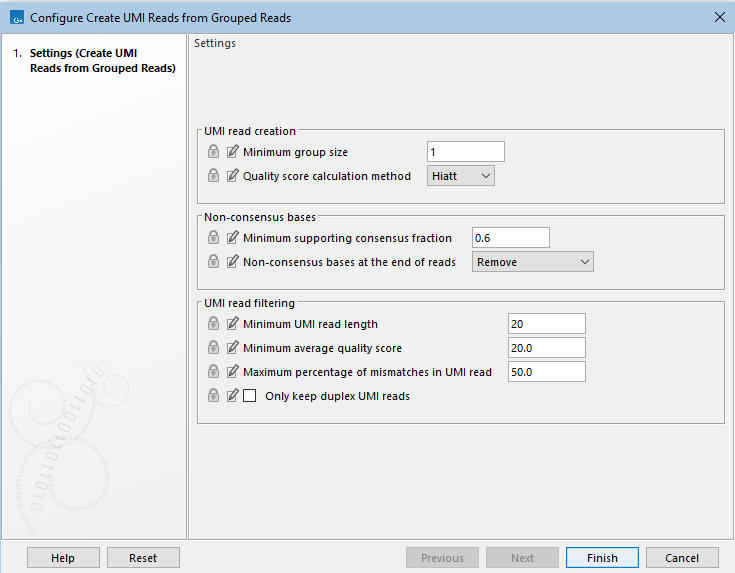Figure 4.5: Settings for the Create UMI Reads from Grouped Reads tool.

• Minimum group size: The tool will only create a UMI read if the number of reads in the UMI is at least "Minimum group size".
• Quality score calculation method: Choose between two methods for computing Q-scores for UMI consensus reads (for more details see Consensus Q-score):
1. Hiatt: The Q-score is calculated following the method described in [Hiatt et al., 2013]. The quality score is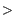of the posterior probability of the consensus base.
2. MAGERI: The Q-score is calculated using a slightly modified version of the method described in [Shugay et al., 2017].

• Non-consensus bases
• Minimum supporting consensus fraction set at 0.6 by default. At each position in the UMI read, the consensus nucleotide is chosen to be the nucleotide with the highest probability of being correct (see the Consensus nucleotide calculation paragraph below). If this probability is higher than "Minimum supporting consensus fraction", a Q score for the consensus nucleotide is calculated. A position in UMI reads that does not have a consensus nucleotide will be considered a N with Q score 0.
• There is a choice between 3 methods of handling non-consensus bases (N with a Q score of 0) that are located at the end of the reads: Remove removes the bases, Keep as unaligned keeps the bases as unaligned ends, and Keep as aligned keeps the bases as aligned bases.

• Minimum UMI read length: UMI shorter than this value will be discarded.
• Minimum average quality score: UMI reads will be discarded, if their average Q-score is lower than "Minimum average quality score".
• Maximum percentage of mismatches in UMI read: UMI reads will be discarded, if more than this percentage of the bases are mismatches.

Click Next to Open or Save the resulting read mapping of UMI reads, i.e., a read mapping of the merged UMI groups. It is also possible to generate a report that will indicate how many reads were ignored and the reason why they were not included in a UMI read. This data will let you verify the found variants, and examine why expected variants were not found.

Consensus nucleotide calculation is performed following the method described in [Hiatt et al., 2013]. The consensus base is chosen so that the posterior probability of the observed read bases is maximized.

In order to maximize the posterior probability of calling a base, i.e.,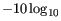where Oi is the observed base at a given position, C the base in question, and where all possible bases are summed up in the denominator, e.g. B=A,T,C,G.

Assuming that the prior for observing any base is equal, i.e., P(A)=P(T)=P(C)=P(G), then the posterior probability is: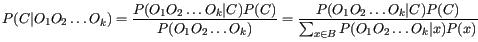And by assuming each read base observation is independent,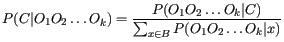To obtain the consensus base we only need to maximize the numerator.

### Consensus Q-score

The Hiatt Q-score isof the probability of making a wrong call, i.e.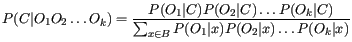which means that the Hiatt Q-score is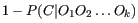Q-scores are capped at 60.

The probabilistic model outlined above and used in the Hiatt Q-score, assumes the only source of errors are independent sequencing errors. While PCR errors are typically rarer than sequencing errors, PCR errors are not independent and they can affect a large fraction of the reads in an UMI group. For this reason, the Hiatt quality scores will often attain the maximum value of 60, even in situations where the reads constituting the UMI group do not unanimously agree on the base call.

The Fixed Ploidy and Low Frequency Variant Detection tools both rely on statistical models for the sequencing error rates, which is estimated for each value of the Q-score and each substitution type, for details see https://resources.qiagenbioinformatics.com/manuals/clcgenomicsworkbench/current/index.php?manual=Variant_Detection_error_model_estimation.html. If most quality scores are 60, the variant callers can not differentiate between, i.e. reads with unanimous agreement and reads without, or between small groups with unanimous agreement and large groups with unanimous agreement.

MAGERI Q-scores does not have a probabilistic interpretation as Hiatt Q-scores, but they a more distributed in the set of possible Q-scores, allowing the variant callers to differentiate between qualities. The MAGERI Q-scores is an adaption of the method described in [Shugay et al., 2017].

First, the frequency, f, of the consensus base is computed, only bases with a Q-score above 25 contribute to the frequency computation. A pseudo-count is applied to the denominator, so that larger groups automatically get higher Q-scores: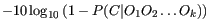where c is the count of the consensus base and n is the total count.

The MAGERI Q-score is then computed as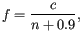This report can be used together with the Combine Reports tool (see http://resources.qiagenbioinformatics.com/manuals/clcgenomicsworkbench/current/index.php?manual=Combine_Reports.html)